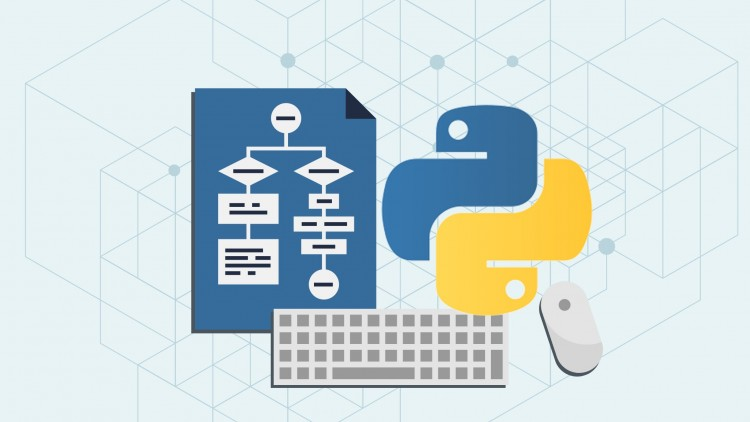# Evaluation of Postfix ExpressionPython Algorithm - Evaluation of Postfix Expression - Data Structure -The Postfix notation is used to represent algebraic expressions.

### Python Algorithm – Evaluation of Postfix Expression:

The Postfix notation is used to represent algebraic expressions. The expressions written in postfix form are evaluated faster compared to infix notation as parenthesis are not required in postfix. We have discussed infix to postfix conversion. In this post, evaluation of postfix expressions is discussed.

### Following is algorithm for evaluation postfix expressions:

Step 1: Create a stack to store operands (or values).
Step 2: Scan the given expression and do following for every scanned element.
…..a) If the element is a number, push it into the stack
…..b) If the element is a operator, pop operands for the operator from stack. Evaluate the operator and push the result back to the stack
Step 3: When the expression is ended, the number in the stack is the final answer

### Example:

Let the given expression be “2 3 1 * + 9 –“. We scan all elements one by one.
1) Scan ‘2’, it’s a number, so push it to stack. Stack contains ‘2’
2) Scan ‘3’, again a number, push it to stack, stack now contains ‘2 3’ (from bottom to top)
3) Scan ‘1’, again a number, push it to stack, stack now contains ‘2 3 1’
4) Scan ‘*’, it’s an operator, pop two operands from stack, apply the * operator on operands, we get 3*1 which results in 3. We push the result ‘3’ to stack. Stack now becomes ‘2 3’.
5) Scan ‘+’, it’s an operator, pop two operands from stack, apply the + operator on operands, we get 3 + 2 which results in 5. We push the result ‘5’ to stack. Stack now becomes ‘5’.
6) Scan ‘9’, it’s a number, we push it to the stack. Stack now becomes ‘5 9’.
7) Scan ‘-‘, it’s an operator, pop two operands from stack, apply the – operator on operands, we get 5 – 9 which results in -4. We push the result ‘-4’ to stack. Stack now becomes ‘-4’.
8) There are no more elements to scan, we return the top element from stack (which is the only element left in stack).

Following is Python implementation of above algorithm.

READ  C Algorithm - Infix to Postfix Conversion using Stack

Python Programming:

``````# Python program to evaluate value of a postfix expression

# Class to convert the expression
class Evaluate:

# Constructor to initialize the class variables
def __init__(self, capacity):
self.top = -1
self.capacity = capacity
# This array is used a stack
self.array = []

# check if the stack is empty
def isEmpty(self):
return True if self.top == -1 else False

# Return the value of the top of the stack
def peek(self):
return self.array[-1]

# Pop the element from the stack
def pop(self):
if not self.isEmpty():
self.top -= 1
return self.array.pop()
else:
return "\$"

# Push the element to the stack
def push(self, op):
self.top += 1
self.array.append(op)

# The main function that converts given infix expression
# to postfix expression
def evaluatePostfix(self, exp):

# Iterate over the expression for conversion
for i in exp:

# If the scanned character is an operand
# (number here) push it to the stack
if i.isdigit():
self.push(i)

# If the scanned character is an operator,
# pop two elements from stack and apply it.
else:
val1 = self.pop()
val2 = self.pop()
self.push(str(eval(val2 + i + val1)))

return int(self.pop())

# Driver program to test above function
exp = "231*+9-"
obj = Evaluate(len(exp))
print "Value of %s is %d" %(exp, obj.evaluatePostfix(exp))

# This code is contributed by Nikhil Kumar Singh(nickzuck_007)``````

### Output:

`Value of 231*+9- is -4`

Time complexity of evaluation algorithm is O(n) where n is number of characters in input expression.

### There are following limitations of above implementation:

1) It supports only 4 binary operators+’, ‘*’, ‘-‘ and ‘/’. It can be extended for more operators by adding more switch cases.
2) The allowed operands are only single digit operands. The program can be extended for multiple digits by adding a separator like space between all elements (operators and operands) of given expression.#### Venkatesan Prabu

Wikitechy Founder, Author, International Speaker, and Job Consultant. My role as the CEO of Wikitechy, I help businesses build their next generation digital platforms and help with their product innovation and growth strategy. I'm a frequent speaker at tech conferences and events.

X